# Chapter 3 Pair of Linear Equation in Two Variables NCERT Exemplar Solutions Exercise 3.4 Class 10 Maths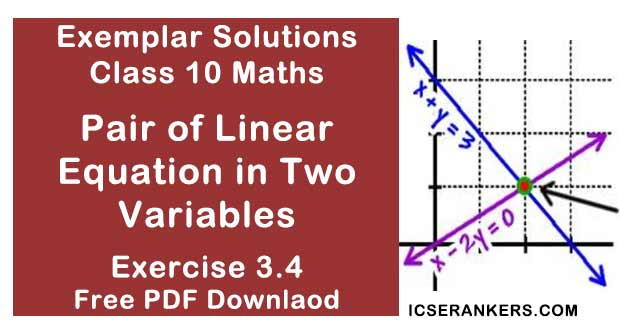Chapter Name NCERT Maths Exemplar Solutions for Chapter 3 Pair of Linear Equation in Two Variables Exercise 3.4 Book Name NCERT Exemplar for Class 10 Maths Other Exercises Exercise 3.1Exercise 3.2Exercise 3.3 Related Study NCERT Solutions for Class 10 Maths

### Exercise 3.4 Solutions

1. Graphically, solve the following pair of equations:
2x + y = 6
2x – y + 2 = 0
Find the ratio of the areas of the two triangles formed by the lines representing these equations with the x-axis and the lines with the y-axis.

Solution

We have
2x + y = 6
2x – y + 2 = 0
For 2x + y – 6 = 0, values of x and y can be,
x = 0, y = 6 and y = 0, x = 3.
Also, for 2x – y + 2 = 0, values of x and y can be,
x = 0, y = 2, and y = 0, x = – 1
Taking A1 and A2 as the areas of triangles ACE and BDE respectively.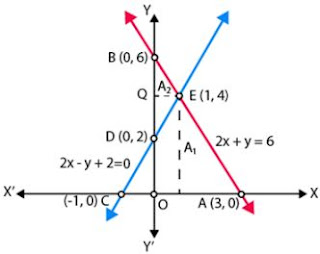If, Area of triangle formed with x -axis = T1
Then,
T1 = Area of △ACE
= 1⁄2 × AC × PE
T1 = 1⁄2 × 4 × 4
= 8
Also,
Area of triangle formed with y – axis = T2
T1 = Area of △BDE
= 1⁄2 × BD × QE
T1 = 1⁄2 × 4 × 1
= 2
Therefore,
T1 :T2 = 8:2
= 4:1
So, the pair of equations intersect graphically at point E (1, 4)
x = 1 and y = 4.

2. Determine, graphically, the vertices of the triangle formed by the lines y = x, 3y = x, x + y = 8

Solution

We have,
y = x ...(i)
3y = x ...(ii)
and x + y = 8 ...(iii)
For line y = x,
x = 0, y = 0
x = 1, y = 1
x = 2, y = 2
Also,
For line x = 3y,
x = 0, y = 0
x = 3, y = 1
x = 6, y = 2
Now, for line x + y = 8
x = 0, y = 8
x = 4, y = 4
x = 8, y = 0
If we plot the graph of above three lines, we will get the following graph,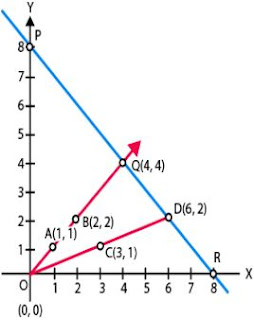We can see that △OQD is formed by these lines.
Therefore, the vertices of the △OQD formed by the given lines are O(0, 0), Q(4, 4) and D(6,2).

3. Draw the graphs of the equations x = 3, x = 5 and 2x – y – 4 = 0. Also find the area of the quadrilateral formed by the lines and the x–axis.

Solution

We have,
x = 3,
x = 5
2x-y-4 = 0.
For line 2x – y – 4 = 0, values of x and y can be,
x = 0, y = -4 and y = 0, x = 2
If we draw the graph, we get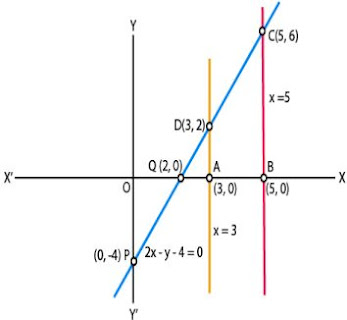So,
AB = OB-OA
= 5-3
= 2
BC = 6
Therefore, quadrilateral ABCD is a trapezium.
Area of quadrilateral ABCD = half × (distance between parallel lines)
= half × (AB) × (AD + BC)
= 8 sq units

4. The cost of 4 pens and 4 pencil boxes is Rs 100. Three times the cost of a pen is Rs 15 more than the cost of a pencil box. Form the pair of linear equations for the above situation. Find the cost of a pen and a pencil box.
Solution
Taking the cost of a pen and a pencil box be Rs x and Rs y respectively.
According to the question,
4x + 4y = 100
x + y = 25 ... (i)
and
3x = y + 15
3x-y = 15 ... (ii)
On adding Equation (i) and (ii), we get,
4x = 40
x = 10
Putting x = 10, in Eq. (i) we get
y = 25-10
= 15
So, the cost of a pen = Rs. 10,and,
The cost of a pencil box = Rs. 15

5. Determine, algebraically, the vertices of the triangle formed by the lines
3x – y = 3
2x – 3y = 2
x + 2y = 8
Solution
3x – y = 3 ...(i)
2x -3y = 2 ...(ii)
x + 2y = 8 ...(iii)
If the equations of the line (i), (ii) and (iii) represent the side of a ∆ABC.
Then, on solving (i) and (ii),
[Multiplying Eq. (i) by 3 and then subtract]
(9x-3y) - (2x-3y) = 9-2
⇒ 7x = 7
⇒ x = 1
Putting,
x =1 in Eq. (i),
We get,
3×1-y = 3
⇒ y = 0
Therefore, the coordinate of point B is (1, 0)
On solving lines (ii) and (iii),
[Multiplying Eq. (iii) by 2 and then subtract]
(2x + 4y) - (2x-3y) = 16 - 2
⇒ 7y = 14
⇒ y = 2
Putting y = 2 in Eq. (iii),
x + 2×2 = 8
⇒ x + 4 = 8
⇒ x = 4
Therefore, the coordinate of point C is (4, 2).
On solving lines (iii) and (i),
[Multiplying in Eq. (i) by 2 and then add]
(6x-2y) + (x + 2y) = 6 + 8
7x = 14
⇒ x = 2
Putting x = 2 in Eq. (i), we get
3×2 – y = 3
⇒ y = 3
Hence, the coordinate of point A is (2, 3).
Therefore, the vertices of the ∆ABC formed by the given lines are:
A (2, 3),
B (1, 0)
C (4, 2).

6. Ankita travels 14 km to her home partly by rickshaw and partly by bus. She takes half an hour if she travels 2 km by rickshaw, and the remaining distance by bus.
Solution
Taking the speed of the rickshaw and the bus x and y km/h, respectively.
As, she has taken time to travel 2 km by rickshaw,
t1 = (2/x) hr
We know that,
Speed = Distance/Time
Time taken to travel remaining distance. (14 – 2) = 12km is,
By bus
t2 = (12/y)hr
By first condition,
t1 + t2 = 1/2
⇒ 2/x + 12/y = 1/2 ...(i)
As, she has taken time to travel 4 km by rickshaw,
t3 = (4/x) hr
and
Time take to travel remaining distance, (14 - 4) = 10 km, by bus,
t4  = (10/y) hr
By second condition,
t3 + t4 = 1⁄2 + 9/60
= 1⁄2 + 3/20
Putting values of t1 and t2 ,
4/x + 10/y = 13/20 ...(ii)
Let(1/x) = u and (1/y) = v
Then Equations, (i) and (ii) becomes
2u + 12v = 1/2 ...(iii)
4u + 10v = 13/20 ...(iv)
[Multiplying Eq. (iii) by 2 and then subtract Eq. (iv)]
(4u + 24v) - (4u + 10v) = 1 - (13/20)
⇒ v = 1/40
Substituting the value of v in Eq. (iii),
2u + 12(1/40) = 1/2
⇒ u = 1/10
⇒ x = 1/u = 10 km/hr
⇒ y = 1/v = 40 km/hr
Therefore, the speed of rickshaw = 10 km/h
And the speed of bus = 40 km/h.

7. A person, rowing at the rate of 5 km/h in still water, takes thrice as much time in going 40 km upstream as in going 40 km downstream. Find the speed of the stream.
Solution
Taking the speed of the stream be x km/hr.
Speed of the boat in still water = 5 km/hr
Speed of the boat upstream = (5 – x)km/hr
Speed of the boat downstream = (5 + x) km/hr
Time taken in rowing 40 km upstream = 40/(5 - x)
Time taken in rowing 40 km downstream = 40/(5 + x)
According to the question, we have
Time taken in  40 km upstream = 3 × Time taken in 40 km downstream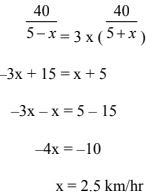Hence, the speed of stream is 2.5 km/hr.

8. A motor boat can travel 30 km upstream and 28 km downstream in 7 hours. It can travel 21 km upstream and return in 5 hours. Find the speed of the boat in still water and the speed of the stream.
Solution
Taking speed of boat in still water = x km/hr
Speed of the stream = y km/hr
Speed of motor boat upstream = (x – y) km/hr
Speed of motor boat downstream = (x + y) km/hr
Case I:
Time taken by motor boat in 30 km upstream = 30/(x - y)
Time taken by motor boat in 28 km downstream = 28(x + y)
30/(x -y) + 28/(x + y) = 7  (Divide by 2)
⇒ 15/(x - y) + 14/(x + y) = 7/2 ...(i)
Case II :
Time taken by motor boat in 21 km upstream = 21/(x - y)
Time taken by motor boat to return 21 km downstream = 21/(x + y)
21/(x - y) + 21/(x + y) = 5  (Divide by 21)
⇒ 1/(x - y) + 1/(x + y) = 5/21  ...(ii)
As both the equations (i) and (ii) are symmetric to (x – y) and (x + y),
We can eliminate either (x–y) or (x+ y).
On solving equation (i) and (ii), we get,
(x – y) = 6 ...(iii)
Putting x – y = 6 in (ii),
x+y = 14 ...(iv)
Solving (iii) and (iv), we get,
x = 10 km/hr
Now,
x + y = 14 [From (iv)]
⇒ 10 + y = 14
y = 4 km/hr
Therefore, the speed of motorboat and stream are 10 km/hr and 4 km/hr respectively.

9. A two-digit number is obtained by either multiplying the sum of the digits by 8 and then subtracting 5 or by multiplying the difference of the digits by 16 and then adding 3. Find the number.
Solution
Let the two digit number be 10x + y
Number = 8(x + y) – 5
10x + y = 8x + 8y – 5
⇒ 10x – 8x + y – 8y = –5
⇒ 2x – 7y = –5 ...(i)
Also,
Number = 16(x – y) + 3 = 10x + y
⇒ 10x + y = 16x – 16y + 3
⇒ –6x + 17y = 3 ...(ii)
Multiplying (i) by 3, we get
6x – 21y = –15 ...(iii)
Adding (iii) and (ii), we have.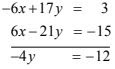So,
y = 3
Now,
2x – 7y = –5 [From (i)]
⇒ 2x – 7(3) = –5
⇒ 2x = –5 + 21
⇒ 2x = 16
⇒ x = 8
So, the number in xy = 83
Therefore, the required number = 83

10. A railway half ticket costs half the full fare, but the reservation charges are the same on a half ticket as on a full ticket. One reserved first class ticket from the station A to B costs Rs 2530. Also, one reserved first class ticket and one reserved first class half ticket from A to B costs Rs 3810. Find the full first-class fare from station A to B, and also the reservation charges for a ticket.
Solution
Taking the cost of full fare from station A to B = ₹ x
And,the reservation charges per ticket = ₹ y
Cost of one full ticket from A to B = ₹ 2530
So,
(1 fare + 1 reservation) charges = ₹ 2530
x + y = 2530  ...(i)
Cost of 1 full and one, half ticket from station A to B = ₹3810
(1 full ticket) + (1/2 ticket) charges = ₹3810
(x + y) + (1/2 fare + reservation) = 3810
⇒ (x + y) + 1/2 x + y = 3810
⇒ 3x/2 + 2y = 3810
⇒ 3x + 4y = 7620  ...(ii)
Multiplying (i), by 3, we get
3x + 3y = 7590 ...(iii)
Subtracting (iii) from (ii), we get
y = 30
Now, x + y = 2530 [From (i)]
x + 30 = 2530 (₹ y = 30)
⇒ x = 2530 – 30
⇒ x = ₹2500
So, full fare and reservation charges of a ticket station A to B are ₹2500 and ₹30
respectively.

11. A shopkeeper sells a saree at 8% profit and a sweater at 10% discount, thereby, getting a sum Rs 1008. If she had sold the saree at 10% profit and the sweater at 8% discount, she would have got Rs 1028. Find the cost price of the saree and the list price (price before discount) of the sweater.

Solution

Taking the cost price of a saree = ₹x
and the list price of sweater = ₹y
Case I:
(S. P. of saree at 8% profit) + (S.P. of a sweater at 10% discount) = ₹1008
108x + 90y = 100800
6x + 5y = 5600 ...(i)
Case II:
(S.P. of saree at 10% profit) + (S.P. of a sweater at 8% discount) = ₹1028
110x + 92y = 102800 ...(ii)
On dividing (ii) by 2,
55x + 46y = 51400 ...(iii)
Multiplying (iii) by 5, we get
275x + 230y = 257000 ...(iv)
Multiplying (i) by 46, we get
276x + 230y = 257600 ...(v)
Subtracting (v) from (iv), we get
–x = –600
⇒ x = ₹ 600
Now,
6x + 5y = 5600 [From (i)]
⇒ 6 × 600 + 5y = 5600 [₹ x = 600]
⇒ 5y = 5600 – 3600
⇒ y = 400
Therefore, the C.P. of a saree and L.P. of sweater are ₹ 600, ₹ 400 respectively.

12. Susan invested certain amount of money in two schemes A and B, which offer interest at the rate of 8% per annum and 9% per annum, respectively. She received Rs 1860 as annual interest. However, had she interchanged the amount of investments in the two schemes, she would have received Rs 20 more as annual interest. How much money did she invest in each scheme?
Solution
Taking, the money invested in scheme A = ₹x
The money invested in scheme B = ₹y
Case I:
Susan invested ₹ x at 8% p.a. + Susan invested ₹y at 9% p.a. = 1860
8x + 9y = 186000 ...(i)
Case II:
Interchanging the amount in schemes A and B, we have
9x + 8y = 188000 ...(ii)
Adding (i) and (ii), we get
x + y = 22000 ...(iii)
Subtracting (i) and (ii),
x – y = 2000 ...(iv)
⇒ x = 12000
Now,
x + y = 22000 [From (iii)]
⇒ y = 22000 – 12000
⇒ y = ₹10,000
Hence, the amount invested in schemes A and B are ₹12000 and ₹ 10,000 respectively.

13. Vijay had some bananas, and he divided them into two lots A and B. He sold the first lot at the rate of Rs 2 for 3 bananas and the second lot at the rate of Re 1 per banana, and got a total of Rs 400. If he had sold the first lot at the rate of Re 1 per banana, and the second lot at the rate of Rs 4 for 5 bananas, his total collection would have been Rs 460. Find the total number of bananas he had.
Solution
Taking the number of bananas in lot A = x
Also, the number of bananas in lot B = y
Case I:
Taking S.P. of 3 bananas of lot A = 2
S.P. of 1 bananas of lot A = 2/3
S.P. of x bananas of lot = 2/3 x
Now, S.P. of 1 banana of lot B = 1
S.P. of y bananas of lot B = y
So,
2x/3 + y = 400
⇒ 2x + 3y = 1200  ...(i)
Case II:
x + 4y/5 = 460
⇒ 5x + 4y = 2300  ...(ii)
Multiplying (i) by 4, we get
8x + 12y = 4800 ...(iii)
Also, multiplying (ii) by 3, we get
15x + 12y = 6900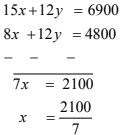So, x = 300
Now,
2x + 3y = 1200 [From (i)]
⇒ 2(300) + 3y = 1200
⇒ 3y = 1200 – 600
⇒ y = 200
So, the total number of bananas = (x + y)
= (300 + 200)
= 500